# 仿真结果共享在Mathematica中的实现

Mathematica在仿真数据上的处理方法是相当优雅的，这个解决方案是InterpolatingFunction。这种函数的表现方式和正常的函数几乎相同，你可以对它求值、求导、求积分等等等等。可能不是对于所有问题，这个方案都能获得最好的结果，但是应该说对于大部分问题这个函数的表现还是比较不错的。Mathematica中有不少函数可以返回InterpolatingFunction，诸如Interpolation, FunctionInterpolation, NDSolve等等。这里举个NDSolve的例子吧！

\begin{equation} m \ddot{x} + c\dot{x} + k x = 0
\end{equation}

\begin{equation} x(0)=1
\end{equation} \begin{equation} \dot{x} (0)=0 \end{equation}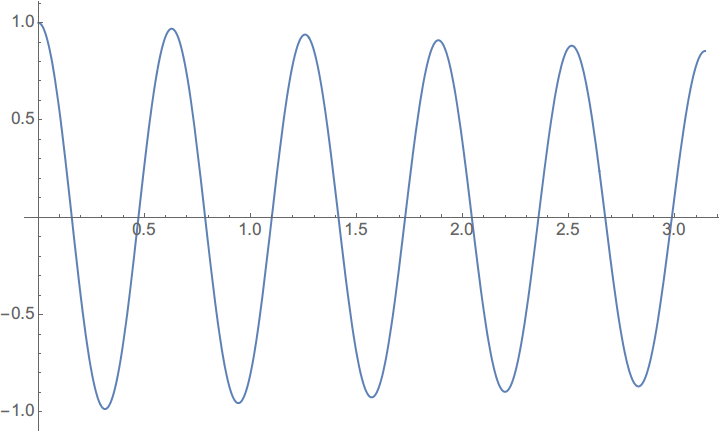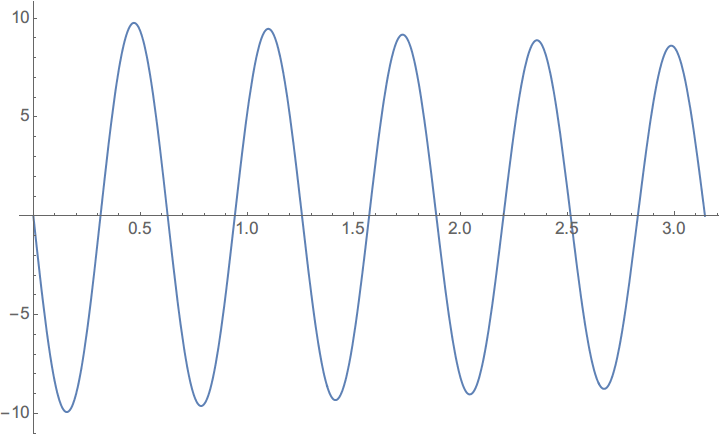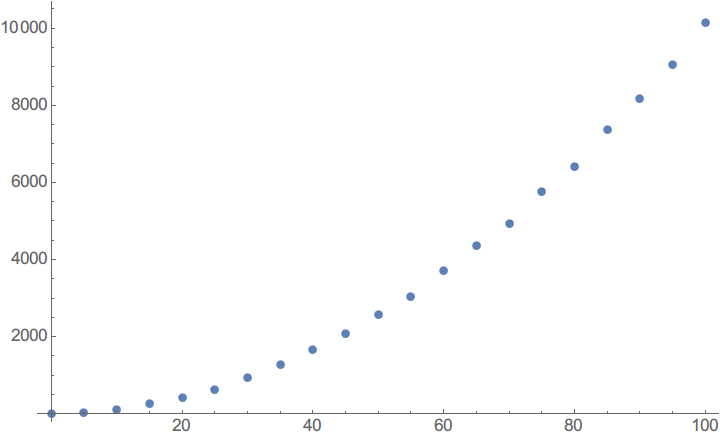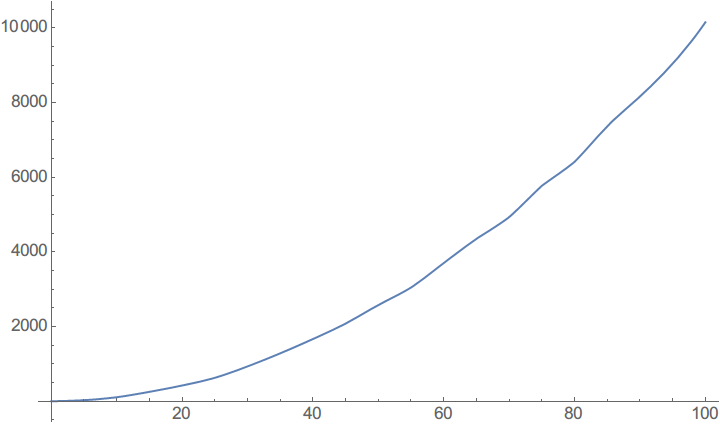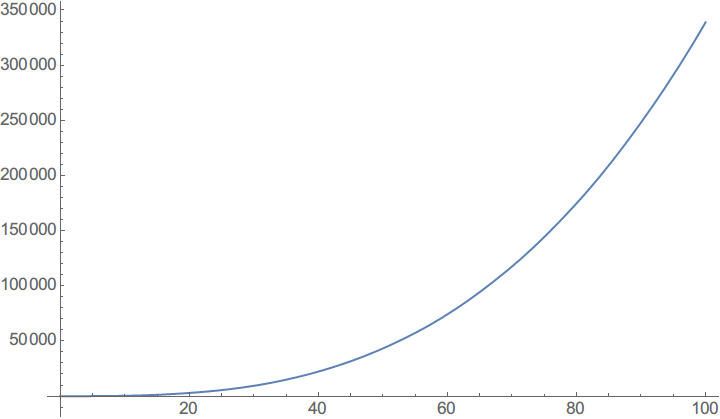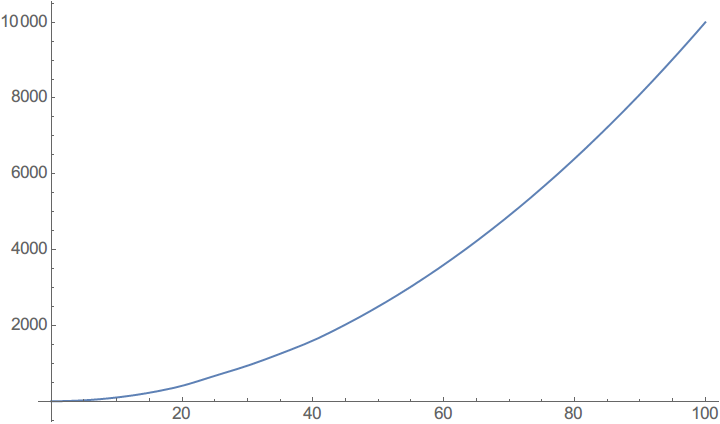## 展开本分类索引